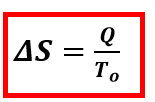# Problem: The element gallium (Ga) freezes at 29.8°C, and its molar enthalpy of fusion is ΔH fus = 5.59kJ/mol.1. When molten gallium solidifies to Ga(s) at its normal melting point, is ΔS positive or negative?2. Calculate the value of ΔS when 70.0g of Ga(l) solidifies at 29.8°C. ΔS = ?

###### FREE Expert Solution

At constant temperature, the change in entropy can be expressed as:Where: Q = heat , T = Temperature

96% (254 ratings)###### Problem Details

The element gallium (Ga) freezes at 29.8°C, and its molar enthalpy of fusion is ΔH fus = 5.59kJ/mol.

1. When molten gallium solidifies to Ga(s) at its normal melting point, is ΔS positive or negative?

2. Calculate the value of ΔS when 70.0g of Ga(l) solidifies at 29.8°C. ΔS = ?